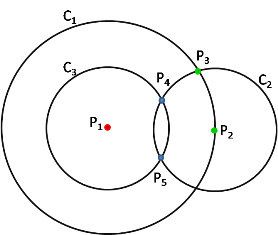# Interesting ProofsAllPages RecentChanges Links to this page Edit this page Search Entry portal Advice For New Users

Theorem 1: Imagine that every point on a plane is coloured either red or green. Then there must be two points of the same colour which are exactly 1 metre apart.

Draw an equilateral triangle with sides 1 metre.

At least two of the vertices of this triangle must be the same colour thereby creating 2 points of the same colour 1 metre apart.

Theorem 2: Imagine that every point on a plane is coloured either red, green or blue. Then there must be two points of the same colour which are exactly 1 metre apart.There exists a red point else the theorem simplifies to Theorem 1 on this page. Label this point $P_1.$

Draw a circle $C_1,$ centre $P_1,$ radius $sqrt{3}$ metres.

Not all points on this circle can be the same colour else a circle $C_2,$ centre $P_2,$ radius 1 metre will produce two points $P_2$ and $P_3$ with the same colour 1 metre apart.

Therefore there exists a non-red point on the circle $C_1.$ Label this point $P_2$ and, without loss of generality, let its colour be green.

Draw circle $C_2,$ centre $P_2,$ radius 1 metre and circle $C_3,$ centre $P_1,$ radius 1 metre.

These circles intersect at $P_4$ and $P_5,$ which are both 1 metre from $P_1$ and $P_2.$

$P_4$ cannot be green or red else the theorem is proved therefore it must be blue. $P_5$ similarly must be blue. However the geometry ensures that $P_4$ and $P_5$ are 1 metre apart so the theorem is proved.

(Here is a lightly rearranged version of this proof that some readers may prefer: Suppose no two points of the same colour are 1m apart. Let P be any point; let Q be $sqrt{3}$ metres away from P. Then we may place points R,S so that together with P,Q they form two equilateral triangles of side 1m, PRS and QRS. From PRS we conclude that R,S are of the two colours that differ from that of P and then from QRS we conclude that Q has the same colour as P. But this works for any point Q that's $sqrt{3}$ metres away from P, and therefore the circle centred at P and of radius $sqrt{3}$ is all the same colour, and it's trivial to find two points on it that are 1m apart.)

Theorem 3: Imagine 6 points joined together either with red lines or green lines. Then there exists a triangle of the same colour.

Take one of the points, P, and see how it is joined to the other five. At least three of the lines must be the same colour, so without loss of generality suppose P is joined with green lines to at least three of the other points.

If any pair of those points is joined with a green line then we have a green triangle.

If no pair of those three points is joined with a green line, then they must all be joined by red lines, and hence we have a red triangle.

There must exist an irrational number raised to an irrational power which gives a rational answer.

 This is an example of the "Heads I win - Tails you lose" method of proof or the application of Archibishop Morton's principle who, as tax collector for Henry VII, reckoned that a house where the occupants lived frugally could afford the taxes from their obvious savings. Alternatively a home with a lavish lifestyle have demonstrated that they have money to spare.
Proof:

Consider $x=sqrt{2}^{sqrt{2}}$ which must be either rational or irrational. If it is rational then we're done. Otherwise $x^sqrt{2}$ is an irrational number to an irrational power, but it equals $sqrt{2}^{sqrt{2}sqrt{2}}=sqrt{2}^2$ which is 2. (As it happens, x is irrational; this is a consequence of the deep and highly nontrivial Gelfond-Schneider theorem.)

Contributions welcome ...